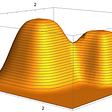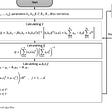# How to perform Roulette wheel and Rank based selection in a genetic algorithm?

• Parents are selected according to their fitness
• The better the chromosomes are, the more chances to be selected they have.
1. Rank selection first ranks the population and then every chromosome receives fitness from this ranking.
2. The worst will have fitness 1, second worst 2 etc. and the best will have fitness N (number of chromosomes in population).
3. After this all the chromosomes have a chance to be selected.
4. Rank-based selection schemes can avoid premature convergence.
5. But can be computationally expensive because it sorts the populations based on fitness value.
6. But this method can lead to slower convergence, because the best chromosomes do not differ so much from other ones.
1. First sort the Fitness value of the Population.
2. Then if the Population number is 10 then give the probability of selection to the Population like 0.1,0.2,0.3,…,1.0 .
3. Then calculate cumulative Fitness and make roulette wheel.
4. And the next steps is same as roulette wheel.
`NewFitness=sort(Fitness);        NewPop=round(rand(PopLength,IndLength));        for i=1:PopLength            for j=1:PopLength                if(NewFitness(i)==Fitness(j))                    NewPop(i,1:IndLength)=CurrentPop(j,1:IndLength);                    break;                end            end        end        CurrentPop=NewPop;        ProbSelection=zeros(PopLength,1);        CumProb=zeros(PopLength,1);        for i=1:PopLength            ProbSelection(i)=i/PopLength;            if i==1                CumProb(i)=ProbSelection(i);            else                CumProb(i)=CumProb(i-1)+ProbSelection(i);            end        end        SelectInd=rand(PopLength,1);        for i=1:PopLength            flag=0;            for j=1:PopLength                if(CumProb(j)<SelectInd(i) && CumProb(j+1)>=SelectInd(i))                    SelectedPop(i,1:IndLength)=CurrentPop(j+1,1:IndLength);                    flag=1;                    break;                end            end            if(flag==0)                SelectedPop(i,1:IndLength)=CurrentPop(1,1:IndLength);            end        end`

--

--

--

## More from Setu Kumar Basak

Everything I do, I do for a reason: to improve myself. Linkedin: https://linkedin.com/in/setu1421/

Love podcasts or audiobooks? Learn on the go with our new app.## Setu Kumar Basak

Everything I do, I do for a reason: to improve myself. Linkedin: https://linkedin.com/in/setu1421/

## My New Machine Learning Book on Stochastic Processes## Improved representation of data, solving the classification problem of imbalanced data## How to perform skull stripping using matlab?## Ensemble learning